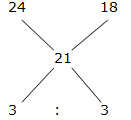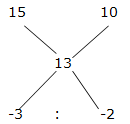# Quantitative Aptitude Questions (Inequality) for RRB Assistant Mains 2018 Day-202

Dear Readers, RRB Assistant is conducting Online Examination for the recruitment of Assistant. To enrich your preparation here we have providing new series of Inequality – Quantitative Aptitude Questions. Candidates those who are appearing in RRB Assistant Mains Exams can practice these Quantitative Aptitude average questions daily and make your preparation effective.

[WpProQuiz 3767]

Click “Start Quiz” to attend these Questions and view Solutions

Directions (Q. 1 – 10): Each question below contains a statement followed by Quantity I and Quantity II. Find both to find the relationship between them. Mark your answer accordingly.

1) Preethi bought two articles A and B at Rs. X and Rs. X + 100 respectively. She sold article A at 15 % profit and article B at 10 % loss, and earned Rs. 30 as profit on the whole deal.

Quantity I:  Find the profit earned by Preethi on selling article A?

Quantity II: Find the loss incurred (in Rs.) by Preethi on selling Article B but the loss % is 15?

a) Quantity I ≤ Quantity II

b) Quantity I ≥ Quantity II

c) Quantity I > Quantity II

d) Quantity I < Quantity II

e) Quantity I = Quantity II or Relation cannot be established

2) Quantity I:  The diagonal of the square is 8√2. The area of the square is?

Quantity II: The perimeter of the square is 28 cm. Then the area of the square is?

a) Quantity I ≤ Quantity II

b) Quantity I ≥ Quantity II

c) Quantity I > Quantity II

d) Quantity I < Quantity II

e) Quantity I = Quantity II or Relation cannot be established

3) A box contains 5 Red balls, 3 white balls, 4 orange balls and 6 black balls.

Quantity I: If two balls are drawn at random, then find the probability that both balls are either Red or Orange?

Quantity II: If four balls are drawn at random, then find the probability that all are of different colours?

a) Quantity I ≤ Quantity II

b) Quantity I ≥ Quantity II

c) Quantity I > Quantity II

d) Quantity I < Quantity II

e) Quantity I = Quantity II or Relation cannot be established

4) Quantity I: 200 m long train can crosses a pole in 5 sec. The speed of the train is?

Quantity II: 250 m long train can crosses a platform of length 200 m in 15 sec. The speed of the train is?

a) Quantity I ≤ Quantity II

b) Quantity I ≥ Quantity II

c) Quantity I > Quantity II

d) Quantity I < Quantity II

e) Quantity I = Quantity II or Relation cannot be established

5) There are 120 students in a class whose average age is 21 years. The average age of the boys in the class is 24 years while the average age of the girls is 18 years?

Quantity I: No of boys in the class is?

Quantity II: No of girls in the class is?

a) Quantity I > Quantity II
b) Quantity I ≥ Quantity II
c) Quantity II > Quantity I
d) Quantity II ≥ Quantity I
e) Quantity I = Quantity II or Relation cannot be established

6) A person invested a sum of Rs. 50000 in two schemes A and B in which it offers simple interest at the rate of 15 % and 10 % respectively. The total interest received from these schemes after 3 years is Rs. 19500.

Quantity I: Sum of money invested in scheme A is?

Quantity II: Sum of money invested in scheme B is?

a) Quantity I ≤ Quantity II

b) Quantity I ≥ Quantity II

c) Quantity I > Quantity II

d) Quantity I < Quantity II

e) Quantity I = Quantity II or Relation can’t be established.

7) 2x × 3y = 108 and 8x = 64

Quantity I: The value of x?

Quantity II: The value of y?

a) Quantity I ≤ Quantity II

b) Quantity I ≥ Quantity II

c) Quantity I > Quantity II

d) Quantity I < Quantity II

e) Quantity I = Quantity II or Relation cannot be established

8) Quantity I: If x2 – 9x – 52 = 0, then the value of x is?

Quantity II: If y2 – 7x – 44 = 0, then the value of y is?

a) Quantity I ≤ Quantity II

b) Quantity I ≥ Quantity II

c) Quantity I > Quantity II

d) Quantity I < Quantity II

e) Quantity I = Quantity II or Relation cannot be established

9) Quantity I: A and B started a business by Rs. 10000 and Rs. 15000 respectively. A invested only 6 months and they divided their shares after a year. The ratio of profit of A and B is 2: 5. How many months B invested the money?

Quantity II: P and Q invested in the ratio of 4: 3. P invested the money for 9 months. The ratio of profit of P and Q is 2: 1. How many months Q invested the money?

a) Quantity I ≤ Quantity II

b) Quantity I ≥ Quantity II

c) Quantity I > Quantity II

d) Quantity I < Quantity II

e) Quantity I = Quantity II or Relation cannot be established

10) Quantity I: The present age of Asha is 12 years more the present age of Rahul. After 5 years the ratio of ages of Rahul and Asha will be 3: 5, and then find the age of Rahul, after 2 years?

Quantity II: The present age of Naveen is twice the present age of Priya. The ratio between the present ages of Gowrish and Priya is 7: 4. If the age of Naveen after 6 years will be 30 years, then find the age of Priya after 3 years?

a) Quantity I ≤ Quantity II

b) Quantity I ≥ Quantity II

c) Quantity I > Quantity II

d) Quantity I < Quantity II

e) Quantity I = Quantity II or Relation cannot be established

Direction (1-10) :

Explanation:

Article A                 Article B

CP      RS. X                     Rs. (x + 100)

SP      Rs. X *(115/100)  Rs. (x + 100)*(90/100)

Now, profit = SP – CP

30 = 115x/100 + (90/100)*(x + 100) – x – x – 100

30 = (115x + 90x + 9000 – 200x – 10000)/100

3000 = 5x – 1000

5x = 4000

X = 800

Quantity I:

Profit earned on Article A

= > 800*(15/100) = Rs. 120

Quantity II:

Loss = 900*(15/100) = Rs. 135

Quantity I < Quantity II

Explanation:

Quantity I:

The diagonal of the square = 8√2

Area of the square = 1/2(d2) = 1/2 (8√2)2

= > (1/2)*64*2 = 64 sq cm

Quantity II:

The perimeter of the square = 4a = 28 cm

Side (a) = 28/4 = 7 cm

Area of the square = (72) = 49 sq cm

Quantity I > Quantity II

Explanation:

Total number of balls = 5 + 3 + 4 + 6 = 18

Quantity I:

n(S) = 18C2

Probability that both the balls are either Red or Orange

n(E) = 5C2 or 4C2

P(E) = n(E)/n(S) = 16/153

Quantity II:

n(S) = 18C4

n(E) = Probability that all the balls are of different colours = 5C1 and 3C1 and 4C1 and 6C1

P(E) = n(E)/n(S) = 2/17

Quantity I < Quantity II

Explanation:

Quantity I:

The speed of the train = 200/5 = 40 m/sec

Quantity II:

The speed of the train = (250 + 200)/15 = 450/15 = 30 m/sec

Quantity I > Quantity II

Explanation:

Boys           girls= > 1 : 1

2’s = 120

1’s = 60

Quantity I: Boys = 60

Quantity II: Girls = 60

Quantity I = Quantity II

Explanation:

Percentage of interest received from these schemes

19500 = (50000*3*r)/100

R = (19500*100)/(50000*3) = 13 %

By the rule of allegation,= > 3 : 2

5’s = 50000

1’s = 10000

Quantity I: Sum of money invested in scheme A= Rs. 30000

Quantity II: Sum of money invested in scheme B = Rs. 20000

Quantity I > Quantity II

Explanation:

8x = 64

8x = 82

X = 2

22 × 3y = 108

3y = 108/4 = 27

3y = 33

Y = 3

Quantity I < Quantity II

Explanation:

Quantity I:

x2 – 9x – 52 = 0

(x – 13) (x + 4) = 0

X = 13, -4

Quantity II:

y2 – 7x – 44 = 0

(y – 11) (y + 4) = 0

Y = 11, -4

Relation cannot be established

Explanation:

Quantity I:

According to the question,

(10000*6)/(15000*x) = (2/5)

60000/15000x = 2/5

60000*5 = 15000x*2

X = (60000*5)/(15000*2)

X = 10 months

Quantity II:

According to the question,

(4*9)/(3x) = (2/1)

36/3x = 2/1

12/x = 2

X = 12/2 = 6

X = 6 months

Quantity I > Quantity II

Explanation:

Quantity I:

Present age of Asha = 12 + Present age of Rahul

After 5 years, the ratio of ages of Rahul and Asha = 3: 5 (3x, 5x)

5x – 3x = 12

2x = 12

X = 6

The age of Rahul, after 2 years = 3x – 5 + 2 = 15 years

Quantity II:

The ratio of present age of Naveen and Priya = 2: 1

The ratio of present age of Gowrish and Priya = 7: 4

The present age of Naveen, Priya and Gowrish = 8: 4: 7

The age of Naveen, after 6 years = 30 years

The present age of Naveen = 24 years

8’s = 24

1’s = 3

The age of Priya after 3 years = 4x + 3 = 15 years

Quantity I = Quantity II

Daily Practice Test Schedule | Good Luck

 Topic Daily Publishing Time Daily News Papers & Editorials 8.00 AM Current Affairs Quiz 9.00 AM Quantitative Aptitude “20-20” 11.00 AM Vocabulary (Based on The Hindu) 12.00 PM General Awareness “20-20” 1.00 PM English Language “20-20” 2.00 PM Reasoning Puzzles & Seating 4.00 PM Daily Current Affairs Updates 5.00 PM Data Interpretation / Application Sums (Topic Wise) 6.00 PM Reasoning Ability “20-20” 7.00 PM English Language (New Pattern Questions) 8.00 PM